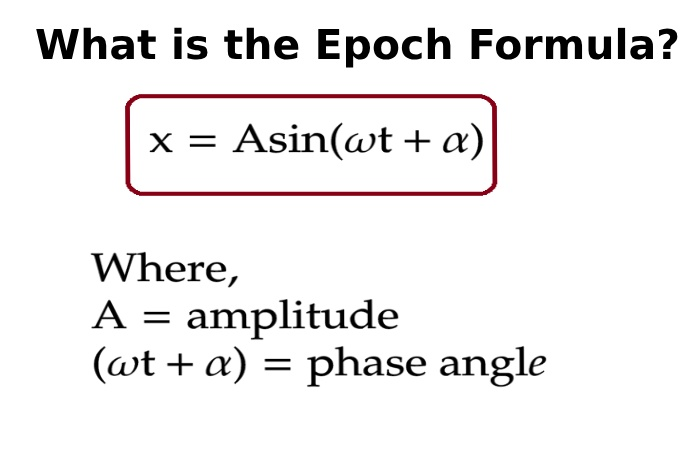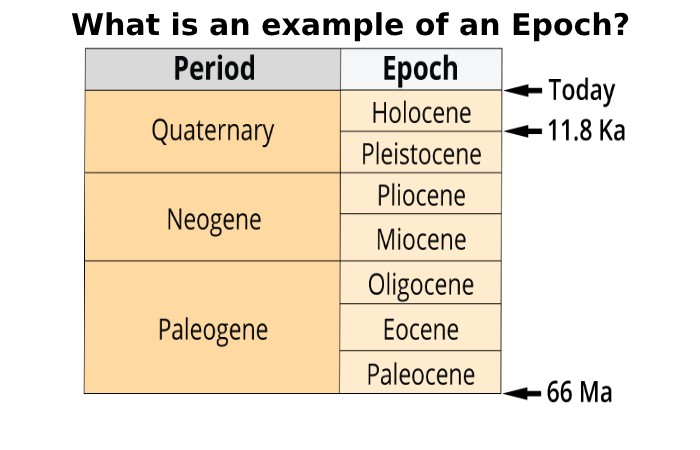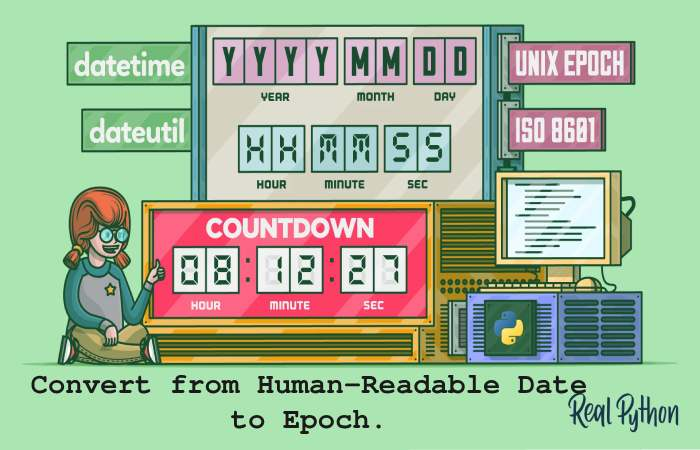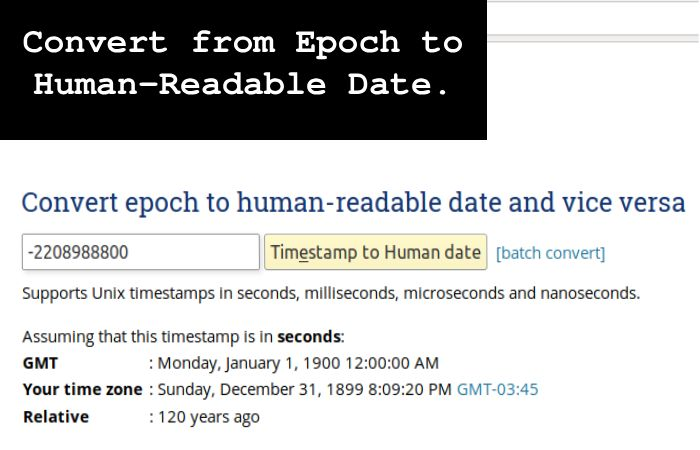An epoch time converter is a tool or program that converts epoch time, also known as Unix time or POSIX time, into a human-readable date and time format. Epoch time is a system for representing time as the number of seconds that have gone then 00:00:00 Coordinated Universal Time (UTC) on January 1, 1970 (not counting leap seconds).

The converter calculates the corresponding year, month, day, hour, minute, and second to convert epoch time to a readable format. This conversion is helpful for programmers, system administrators, and anyone working with timestamped data, as it makes time information more accessible and understandable.

To convert epoch time to a human-readable date and time format, follow these steps:

1. Obtain the epoch time value you want to convert.
2. Use a programming language or a working converter tool that supports epoch time conversions.
3. Divide the epoch time by 1,000 if it’s in milliseconds or leave it as-is for seconds since epoch time is usually represented in one of these units.
4. Use the converted value to create a date and time. For example, you can use the Date Time library in Python to achieve this.

Import Datetime

epoch_time = 1632055200  # Replace with your epoch time

1. Adjust the format to suit your needs (e.g., ‘YYYY-MM-DD HH:MM: SS’).

The result will be a human-readable representation of the epoch time in the specified format.

## What is the Epoch Formula?The epoch formula is a mathematical representation used to calculate epoch time, known as Unix or POSIX time. It defines the number of seconds that have passed since 00:00:00 Coordinated Universal Time (UTC) on January 1, 1970 (excluding leap seconds).

The formula is:

Epoch Time (in seconds) = Total Seconds between a Given Time and January 1, 1970 (UTC)

It calculates the difference between the given time and the reference point, which is the start of the Unix epoch. The result is a timestamp representing the time in seconds since that epoch, making it a standardized way to represent time across different computer systems and programming languages.

## Why is it called Epoch Time?

Epoch time is named after the term “epoch” in the context of astronomy and geology, where it refers to a specific reference point in time from which other time measurements are made. In computing, the choice of January 1, 1970, as the starting point for epoch time was adopted by Unix developers as a practical and convenient reference point for measuring time in seconds.

This date was selected because it preceded the creation of the Unix operating system, allowing for negative time values to represent dates before 1970. This standardized system became Unix or epoch time, serving as a standard timestamp format across computing platforms.

## What is an example of an Epoch?An example of an epoch in computing is the Unix epoch, widely used to represent time. The Unix epoch refers to the moment when time started for the Unix operating system and is set on January 1, 1970, at 00:00:00 Coordinated Universal Time (UTC).

At that instant, the epoch time defining as 0 seconds. Since then, the epoch time has continuously increased by one second for each passing second. For instance, if you have an epoch time value of 1632055200, it represents September 19, 2021, at 14:00:00 UTC (or a corresponding time in the local timezone). Epoch time provides a standardized way to express time across different computer systems and programming languages.

## Epoch dates used for the start and end of the year/month/day

The epoch time for the start of the year, month, and day can be calculated as follows:

• Start of the Year (January 1): The epoch time for the start of January 1, 1970, is 0 seconds. It is the reference point for Unix epoch time.
• Start of a Month: To find the epoch time for the start of a specific month and year, you’d calculate the number of seconds that have elapsed between that date and January 1, 1970. For example, the epoch time for the start of February 1, 2023, is 1674988800 seconds.
• Start of a Day: Similarly, to find the epoch time for the start of a specific day, you’d calculate the seconds between that date and the Unix epoch. For example, the epoch time for the start of September 19, 2023, would be 1692556800 seconds.

These epoch times widely used in computing and programming to accurately represent and calculate time intervals.

## How do we get the Current Epoch Time?

You can use system libraries or functions to obtain the current epoch time in most programming languages. For example, in Python, you can use the time module:

Import Time

current_epoch_time = int(time.time())

In this code, time.time() returns the current time in seconds since the Unix epoch (January 1, 1970), and int() is used to convert it into an integer to remove the decimal part. Similar functions or methods exist in other languages, such as time() in JavaScript and System.currentTimeMillis() in Java. This epoch time is valuable for various applications like timestamping, measuring time intervals, and synchronization.

## Convert from Human-Readable Date to Epoch.

A human-readable date can convert to epoch time using programming languages and libraries. For instance, in Python, you can use the date time module:From Date Time – Import Date Time

date_string = “2023-09-19 14:30:00”  # Replace with your desired date and time

datetime_object = datetime.strptime(date_string, ‘%Y-%m-%d %H:%M:%S’)

epoch_time = int(datetime_object.timestamp())

This code uses strptime to parse the human-readable date string into a DateTime object, and timestamp() extracts the corresponding epoch time in seconds. Similar functions or methods are available in other programming languages like JavaScript and Java. This conversion is useful for data processing, event scheduling, and timestamp manipulation.

## Convert from Epoch to Human-Readable Date.You can use programming languages and libraries to convert epoch time (seconds since January 1, 1970) to a human-readable date. In Python, for instance, you can do this:

Import Datetime

epoch_time = 1632055200  # Replace with your epoch time

In this code, utcfromtimestamp converts the epoch time into a datetime object in UTC and strftime formats it into a human-readable date and time string. Similar functions exist in other languages like JavaScript and Java. This conversion is valuable for displaying timestamps, scheduling tasks, and handling time-related data in a user-friendly way.

## How many Epochs in a Day?

There are 86,400 seconds in a day because 60 seconds in a minute, 60 minutes in an hour, and 24 hours in a day (60 x 60 x 24 = 86,400). Since epoch time measures in seconds since January 1, 1970, there are also 86,400 epochs daily.

It means that the epoch time value increases by one every second, so each day has 86,400 different epoch time values, each representing a unique second within that day. Epoch time is a precise way to measure time intervals and is widely used in computing and programming for timestamping and date calculations.

### Conclusion

Epoch time, or Unix time or POSIX time, is a system for tracking time in computing and represents the number of seconds that have gone since January 1, 1970, at 00:00:00 UTC (Coordinated Universal Time). It’s a way to represent time as a single integer, which makes it convenient for various computer systems to work with timestamps.

You can use programming languages like Python, JavaScript, or online epoch time converters to convert epoch time to a more human-readable date and time format.

Related Searches –
Epoch time now
Epoch time converter excel
Unix time converter
Epoch time milliseconds
Timestamp converter
Timestamp to date
Unix epoch time
Milliseconds to date